Function Repository Resource:

# VennGraphPlot

Visualize the intersections of overlapping sets

Contributed by: Michael Sollami
 ResourceFunction["VennGraphPlot"][{set1, set2, …}] plots a graph showing the number of shared elements among the sets.

## Details and Options

The graph visualizes the elements shared among the sets in what's also known as a Venn lattice.
ResourceFunction["VennGraphPlot"] takes the following options:
 "Colors" Automatic colors to use for the primary sets GraphLayout Automatic the embedding used in laying out the graph "Labels" Automatic labels to use for the sets "Orders" All display the n–way intersections in the graph "TooltipSamples" 10 the number of samples from each set to display in the tooltip "TooltipRandomize" True whether to show random samples in tooltip

## Examples

### Basic Examples (3)

Visualize the intersections of three sets:

 In:=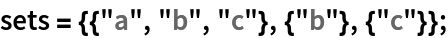In:=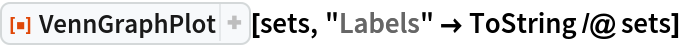Out=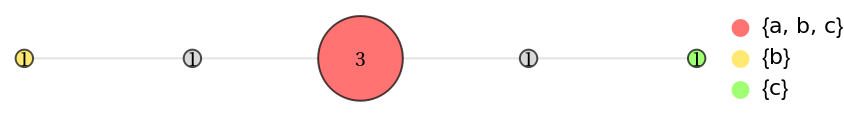Visualize the pairwise intersections of sets:

 In:=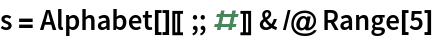Out=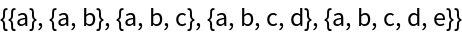In:=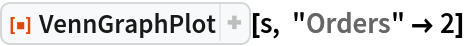Out=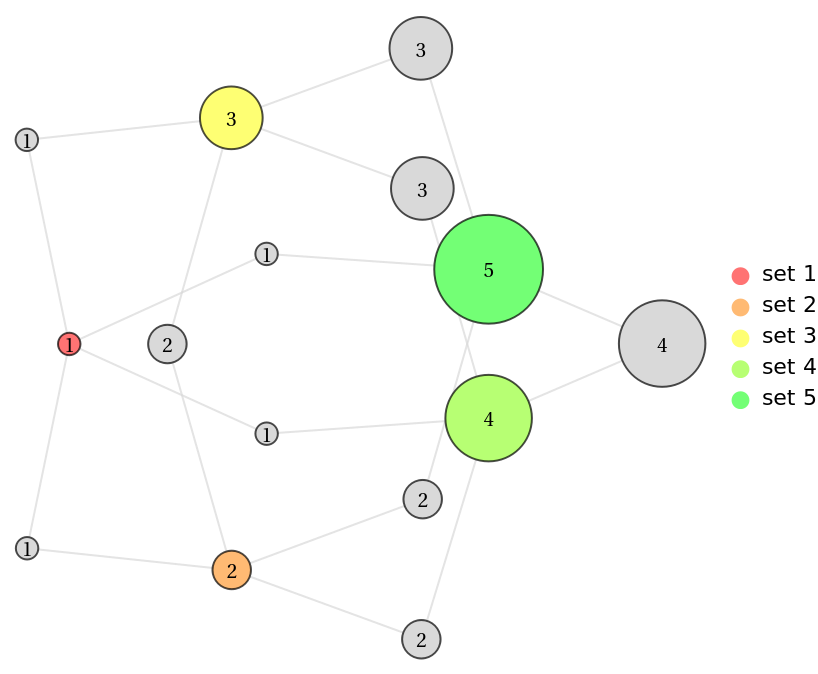Plot a Venn graph with different intersection orders:

 In:=Out=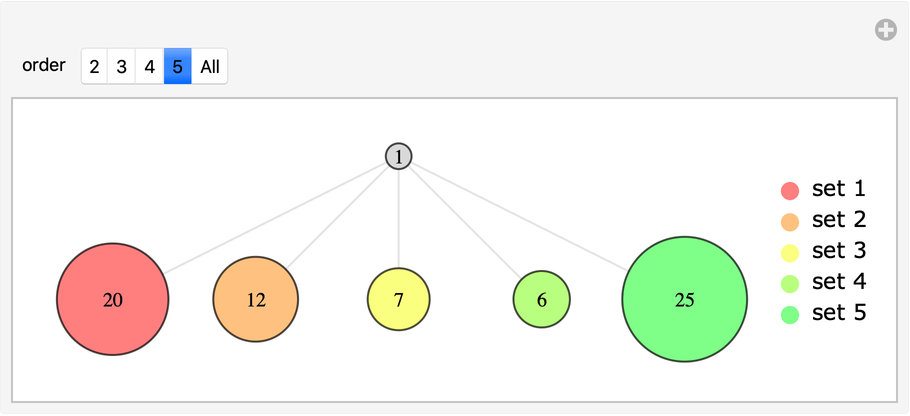### Options (2)

You can change the colors used for the sets node with the "Colors" option:

 In:=Out=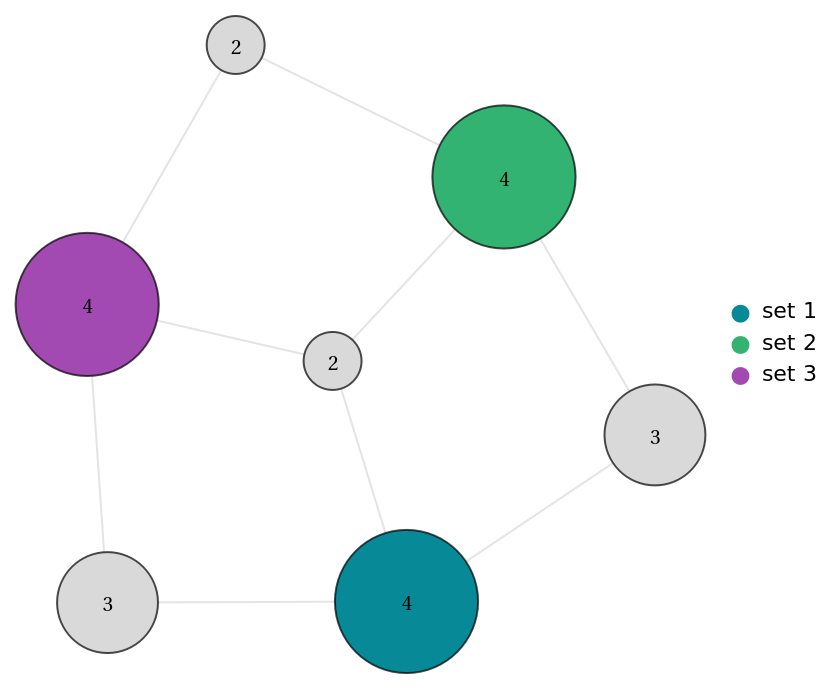You can change the layout used with the GraphLayout option:

 In:=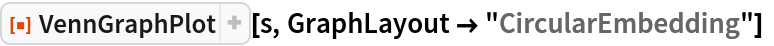Out=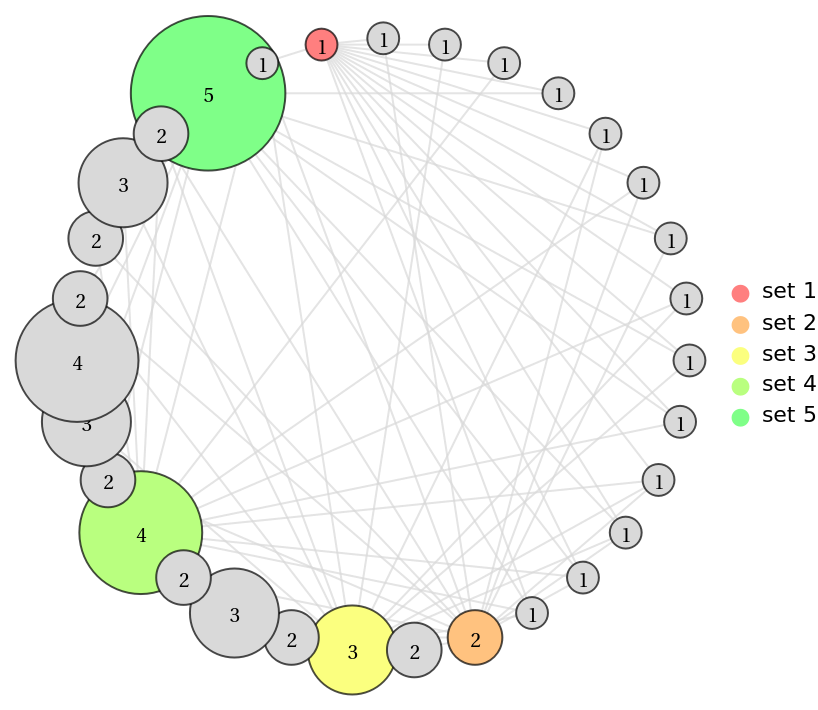### Applications (1)

Show the size of the shared memberships between four political blocs:

 In:=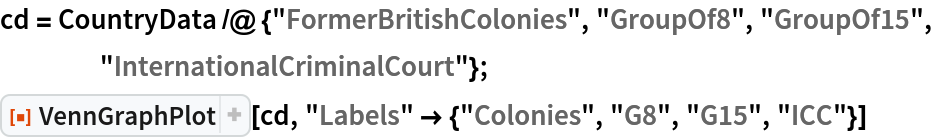Out=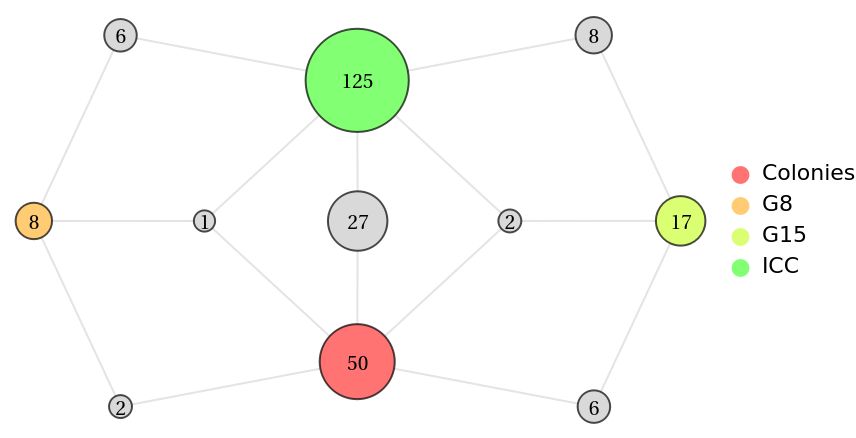### Possible Issues (2)

There is no lattice for totally independent sets :

 In:=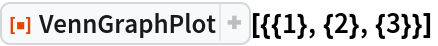Out=The number of intersections can increase exponentially:

 In:=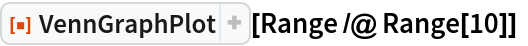Out=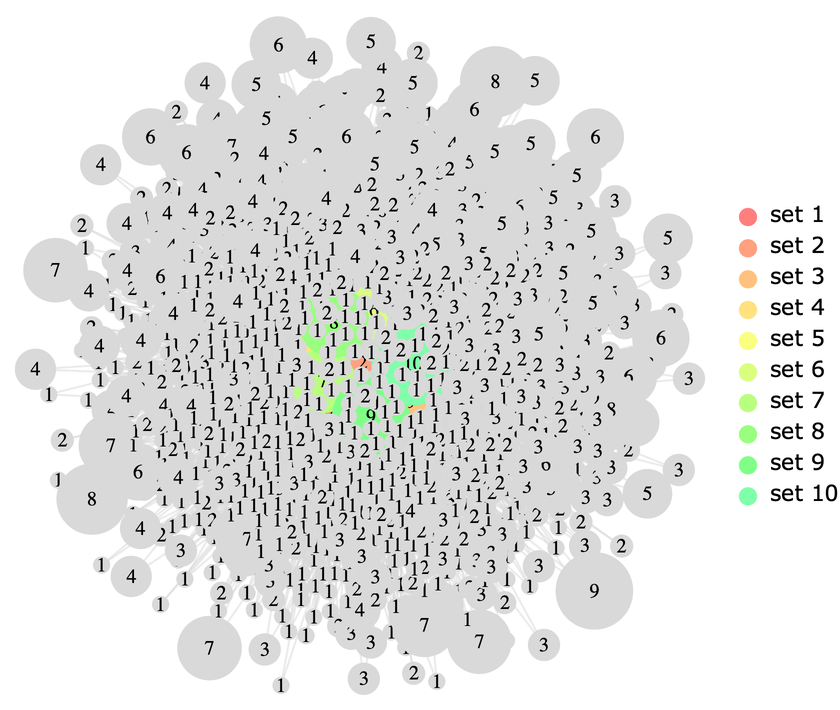Michael Sollami

## Version History

• 2.0.0 – 17 March 2020
• 1.0.0 – 26 February 2020